Kernel Density Estimates

A weighted univariate kernel density estimate involves a variable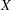and a weight variable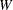. Let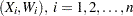, denote a sample ofandof size. The weighted kernel density estimate of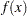, the density of, is as follows: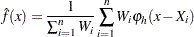whereis the bandwidth and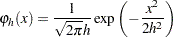is the standard normal density rescaled by the bandwidth. If
and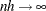, then the optimal bandwidth is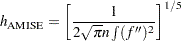This optimal value is unknown, and so approximations methods are required. For a derivation and discussion of these results, refer to Silverman (1986, Chapter 3) and Jones, Marron, and Sheather (1996).

For the bivariate case, let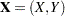be a bivariate random element taking values inwith joint density function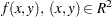and let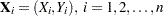, be a sample of sizedrawn from this distribution. The kernel density estimate of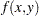based on this sample is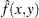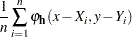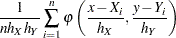where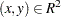,
and
are the bandwidths, and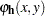is the rescaled normal density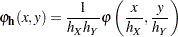where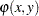is the standard normal density function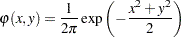Under mild regularity assumptions about, the mean integrated squared error (MISE) ofis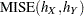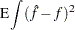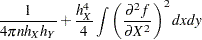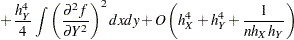as
,
and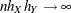.

Now set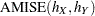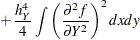which is the asymptotic mean integrated squared error (AMISE). For fixed, this has a minimum at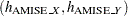defined as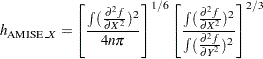and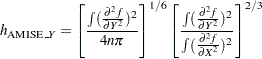These are the optimal asymptotic bandwidths in the sense that they minimize MISE. However, as in the univariate case, these expressions contain the second derivatives of the unknown density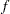being estimated, and so approximations are required. Refer to Wand and Jones (1993) for further details.